# 网格和马丁格尔交易系统中的机器学习。 您敢为其打赌吗？

### 成交贴标签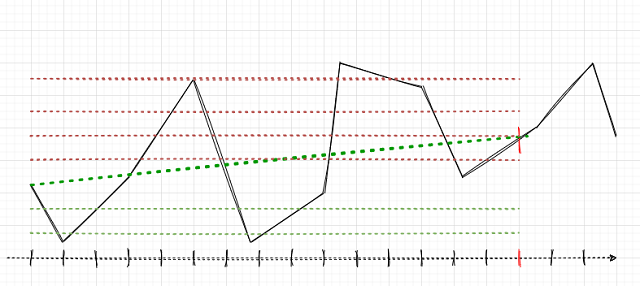• 依据最大总利润进行选择。 如果“卖出”网格产生更多的利润，则为该网格贴标签。
• 在持单数量和总利润之间进行加权选择。 如果网格中每笔持单的平均利润高于逆向的平均利润，则选择该边。
• 依据已触发订单的最大数量进行选择。 由于所期望的机器人应遵照网格交易，该选项看起来很合理。 如果已触发订单数量最大，且总仓位处于获利状态，则选择此侧。 这一侧代表网格的方向（卖出或买入）。

### 在代码中为成交贴标签

```def add_labels(dataset, min, max):
labels = []
for i in range(dataset.shape-max):
rand = random.randint(min, max)
curr_pr = dataset['close'][i]
future_pr = dataset['close'][i + rand]

if future_pr + MARKUP < curr_pr:
labels.append(1.0)
elif future_pr - MARKUP > curr_pr:
labels.append(0.0)
else:
labels.append(2.0)
dataset = dataset.iloc[:len(labels)].copy()
dataset['labels'] = labels
dataset = dataset.dropna()
dataset = dataset.drop(
dataset[dataset.labels == 2].index).reset_index(drop=True)
return dataset
```

```GRID_SIZE = 10
GRID_DISTANCES = np.full(GRID_SIZE, 0.00200)
GRID_COEFFICIENTS = np.linspace(1, 3, num= GRID_SIZE)
```

GRID_SIZE 变量里包含双向订单的数量。

GRID_DISTANCES 设置订单之间的距离。 距离可以是固定的，亦或可变的（对于所有订单而言各有不同）。 这将有助于提升交易系统的灵活性。

GRID_COEFFICIENTS 变量包含每笔订单的手数乘数。 如果它们是常数，则系统将创建规则的网格。 如果手数不同，那么它会是马丁格尔或逆马丁格尔，或任何其他运用不同手数乘数的网格名称。

• np.full 用指定数量的相同值填充数组
• np.linspace 用指定数量的值填充数组，其值均匀地分布在两个实数之间。 在上面的示例中，GRID_COEFFICIENTS 将包含以下内容。

```array([1.        , 1.22222222, 1.44444444, 1.66666667, 1.88888889,
2.11111111, 2.33333333, 2.55555556, 2.77777778, 3.        ])
```

```def add_labels(dataset, min, max, distances, coefficients):
labels = []
for i in range(dataset.shape-max):
rand = random.randint(min, max)
all_pr = dataset['close'][i:i + rand + 1]

grid_stats = {'up_range': all_pr - all_pr.min(),
'dwn_range': all_pr.max() - all_pr,
'up_state': 0,
'dwn_state': 0,
'up_orders': 0,
'dwn_orders': 0,
'up_profit': all_pr[-1] - all_pr - MARKUP,
'dwn_profit': all_pr - all_pr[-1] - MARKUP
}

for i in np.nditer(distances):
if grid_stats['up_state'] + i <= grid_stats['up_range']:
grid_stats['up_state'] += i
grid_stats['up_orders'] += 1
grid_stats['up_profit'] += (all_pr[-1] - all_pr + grid_stats['up_state']) \
* coefficients[int(grid_stats['up_orders']-1)]
grid_stats['up_profit'] -= MARKUP * coefficients[int(grid_stats['up_orders']-1)]

if grid_stats['dwn_state'] + i <= grid_stats['dwn_range']:
grid_stats['dwn_state'] += i
grid_stats['dwn_orders'] += 1
grid_stats['dwn_profit'] += (all_pr - all_pr[-1] + grid_stats['dwn_state']) \
* coefficients[int(grid_stats['dwn_orders']-1)]
grid_stats['dwn_profit'] -= MARKUP * coefficients[int(grid_stats['dwn_orders']-1)]

if grid_stats['up_profit'] > grid_stats['dwn_profit'] and grid_stats['up_profit'] > 0:
labels.append(0.0)
continue
elif grid_stats['dwn_profit'] > 0:
labels.append(1.0)
continue

labels.append(2.0)

dataset = dataset.iloc[:len(labels)].copy()
dataset['labels'] = labels
dataset = dataset.dropna()
dataset = dataset.drop(
dataset[dataset.labels == 2].index).reset_index(drop=True)
return dataset
```

all_pr 变量包含从当前到未来的价格。 需要计算网格本身。 为了构建网格，我们想知道从第一根到最后一根柱线的价格范围。 这些值包含在 “up_range” 和 “dwn_range” 字典条目当中。 变量 “up_profit” 和 “dwn_profit” 将包含当前历史片段中的 “买入” 或 “卖出” 网格所获得的最终利润。 这些数值的初始值，来自最初开仓的一笔成交中获得的利润。 然后，如果网格挂单被触发，所有开单成交均会汇总。

```grid_stats['up_profit'] += (all_pr[-1] - all_pr + grid_stats['up_state']) \
* coefficients[int(grid_stats['up_orders']-1)]
grid_stats['up_profit'] -= MARKUP * coefficients[int(grid_stats['up_orders']-1)]
```

### 升级测试器以便能操控订单网格

```def tester(dataset, markup, distances, coefficients, plot=False):
last_deal = int(2)
all_pr = np.array([])
report = [0.0]
for i in range(dataset.shape):
pred = dataset['labels'][i]
all_pr = np.append(all_pr, dataset['close'][i])

if last_deal == 2:
last_deal = 0 if pred <= 0.5 else 1
continue

if last_deal == 0 and pred > 0.5:
last_deal = 1
up_range = all_pr - all_pr.min()
up_state = 0
up_orders = 0
up_profit = (all_pr[-1] - all_pr) - markup
report.append(report[-1] + up_profit)
up_profit = 0
for d in np.nditer(distances):
if up_state + d <= up_range:
up_state += d
up_orders += 1
up_profit += (all_pr[-1] - all_pr + up_state) \
* coefficients[int(up_orders-1)]
up_profit -= markup * coefficients[int(up_orders-1)]
report.append(report[-1] + up_profit)
up_profit = 0
all_pr = np.array([dataset['close'][i]])
continue

if last_deal == 1 and pred < 0.5:
last_deal = 0
dwn_range = all_pr.max() - all_pr
dwn_state = 0
dwn_orders = 0
dwn_profit = (all_pr - all_pr[-1]) - markup
report.append(report[-1] + dwn_profit)
dwn_profit = 0
for d in np.nditer(distances):
if dwn_state + d <= dwn_range:
dwn_state += d
dwn_orders += 1
dwn_profit += (all_pr + dwn_state - all_pr[-1]) \
* coefficients[int(dwn_orders-1)]
dwn_profit -= markup * coefficients[int(dwn_orders-1)]
report.append(report[-1] + dwn_profit)
dwn_profit = 0
all_pr = np.array([dataset['close'][i]])
continue

y = np.array(report).reshape(-1, 1)
X = np.arange(len(report)).reshape(-1, 1)
lr = LinearRegression()
lr.fit(X, y)

l = lr.coef_
if l >= 0:
l = 1
else:
l = -1

if(plot):
plt.figure(figsize=(12,7))
plt.plot(report)
plt.plot(lr.predict(X))
plt.title("Strategy performance")
plt.ylabel("cumulative profit in pips")
plt.show()

return lr.score(X, y) * l
```

### 测试操控订单网格的新方法

```# Get prices and labels and test it

pr = get_prices(START_DATE, END_DATE)
pr = add_labels(pr, 15, 15, GRID_DISTANCES, GRID_COEFFICIENTS)
tester(pr, MARKUP, GRID_DISTANCES, GRID_COEFFICIENTS, plot=True)
```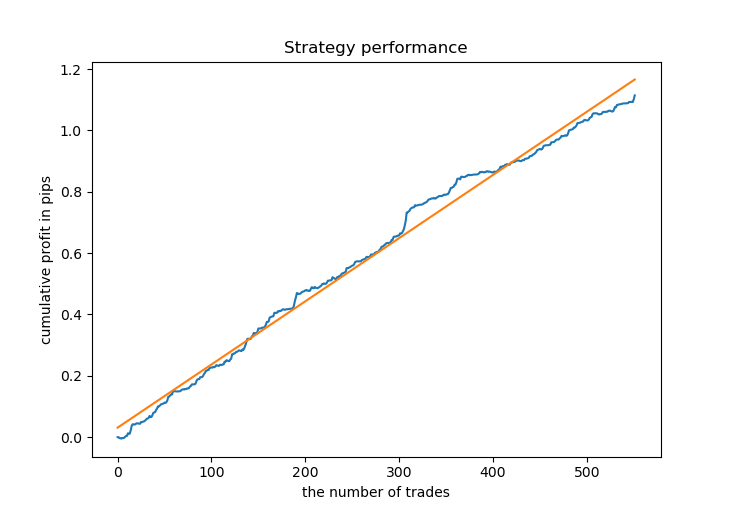```# Learn and test CatBoost model

gmm = mixture.GaussianMixture(
n_components=N_COMPONENTS, covariance_type='full', n_init=1).fit(pr[pr.columns[1:]])
res = []
for i in range(10):
res.append(brute_force(10000))
print('Iteration: ', i, 'R^2: ', res[-1])
res.sort()
test_model(res[-1])
```

```Iteration:  0 R^2:  0.8719436661855786
Iteration:  1 R^2:  0.912006346274096
Iteration:  2 R^2:  0.9532278725035132
Iteration:  3 R^2:  0.900845571741786
Iteration:  4 R^2:  0.9651728908727953
Iteration:  5 R^2:  0.966531822300101
Iteration:  6 R^2:  0.9688263099200539
Iteration:  7 R^2:  0.8789927823514787
Iteration:  8 R^2:  0.6084261786804662
Iteration:  9 R^2:  0.884741078512629
```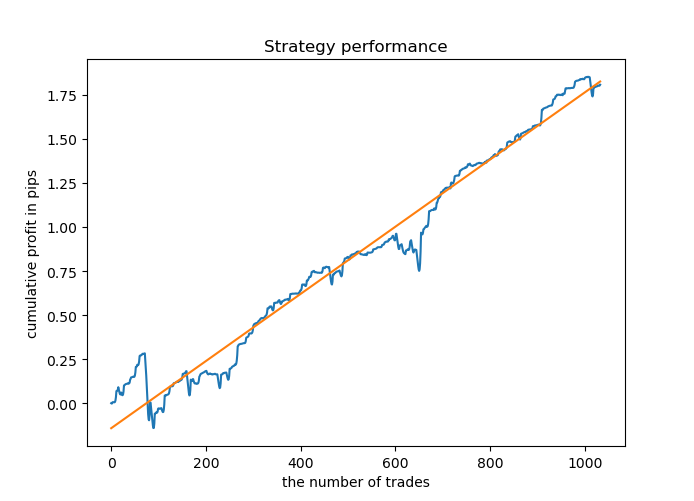### 把 CatBoost 模型导出到 MQL5

`export_model_to_MQL_code(res[-1])`

```def export_model_to_MQL_code(model):
model.save_model('catmodel.h',
format="cpp",
export_parameters=None,
pool=None)

code = '#include <Math\Stat\Math.mqh>'
code += '\n'
code += 'int MAs[' + str(len(MA_PERIODS)) + \
'] = {' + ','.join(map(str, MA_PERIODS)) + '};'
code += '\n'
code += 'int grid_size = ' + str(GRID_SIZE) + ';'
code += '\n'
code += 'double grid_distances[' + str(len(GRID_DISTANCES)) + \
'] = {' + ','.join(map(str, GRID_DISTANCES)) + '};'
code += '\n'
code += 'double grid_coefficients[' + str(len(GRID_COEFFICIENTS)) + \
'] = {' + ','.join(map(str, GRID_COEFFICIENTS)) + '};'
code += '\n'

# get features
code += 'void fill_arays( double &features[]) {\n'
code += '   double pr[], ret[];\n'
code += '   ArrayResize(ret, 1);\n'
code += '   for(int i=ArraySize(MAs)-1; i>=0; i--) {\n'
code += '       CopyClose(NULL,PERIOD_CURRENT,1,MAs[i],pr);\n'
code += '       double mean = MathMean(pr);\n'
code += '       ret = pr[MAs[i]-1] - mean;\n'
code += '       ArrayInsert(features, ret, ArraySize(features), 0, WHOLE_ARRAY); }\n'
code += '   ArraySetAsSeries(features, true);\n'
code += '}\n\n'

code += 'double catboost_model' + '(const double &features[]) { \n'
code += '    '
with open('catmodel.h', 'r') as file:
code += data[data.find("unsigned int TreeDepth")
:data.find("double Scale = 1;")]
code += '\n\n'
code += 'return ' + \
'ApplyCatboostModel(features, TreeDepth, TreeSplits , BorderCounts, Borders, LeafValues); } \n\n'

code += 'double ApplyCatboostModel(const double &features[],uint &TreeDepth_[],uint &TreeSplits_[],uint &BorderCounts_[],float &Borders_[],double &LeafValues_[]) {\n\
uint FloatFeatureCount=ArrayRange(BorderCounts_,0);\n\
uint BinaryFeatureCount=ArrayRange(Borders_,0);\n\
uint TreeCount=ArrayRange(TreeDepth_,0);\n\
bool     binaryFeatures[];\n\
ArrayResize(binaryFeatures,BinaryFeatureCount);\n\
uint binFeatureIndex=0;\n\
for(uint i=0; i<FloatFeatureCount; i++) {\n\
for(uint j=0; j<BorderCounts_[i]; j++) {\n\
binaryFeatures[binFeatureIndex]=features[i]>Borders_[binFeatureIndex];\n\
binFeatureIndex++;\n\
}\n\
}\n\
double result=0.0;\n\
uint treeSplitsPtr=0;\n\
uint leafValuesForCurrentTreePtr=0;\n\
for(uint treeId=0; treeId<TreeCount; treeId++) {\n\
uint currentTreeDepth=TreeDepth_[treeId];\n\
uint index=0;\n\
for(uint depth=0; depth<currentTreeDepth; depth++) {\n\
index|=(binaryFeatures[TreeSplits_[treeSplitsPtr+depth]]<<depth);\n\
}\n\
result+=LeafValues_[leafValuesForCurrentTreePtr+index];\n\
treeSplitsPtr+=currentTreeDepth;\n\
leafValuesForCurrentTreePtr+=(1<<currentTreeDepth);\n\
}\n\
return 1.0/(1.0+MathPow(M_E,-result));\n\
}'

str(SYMBOL) + '_cat_model_martin' + '.mqh', "w")
file.write(code)
file.close()
print('The file ' + 'cat_model' + '.mqh ' + 'has been written to disc')
```

```#include <Math\Stat\Math.mqh>
int MAs = {5,25,55,75,100,125,150,200,250,300,350,400,450,500};
int grid_size = 10;
double grid_distances = {0.003,0.0035555555555555557,0.004111111111111111,0.004666666666666666,0.005222222222222222,
0.0057777777777777775,0.006333333333333333,0.006888888888888889,0.0074444444444444445,0.008};
double grid_coefficients = {1.0,1.4444444444444444,1.8888888888888888,2.333333333333333,
2.7777777777777777,3.2222222222222223,3.6666666666666665,4.111111111111111,4.555555555555555,5.0};
void fill_arays( double &features[]) {
double pr[], ret[];
ArrayResize(ret, 1);
for(int i=ArraySize(MAs)-1; i>=0; i--) {
CopyClose(NULL,PERIOD_CURRENT,1,MAs[i],pr);
double mean = MathMean(pr);
ret = pr[MAs[i]-1] - mean;
ArrayInsert(features, ret, ArraySize(features), 0, WHOLE_ARRAY); }
ArraySetAsSeries(features, true);
}
```

`#include <EURUSD_cat_model_martin.mqh>`

```void OnTick() {
//---
if(!isNewBar()) return;
TimeToStruct(TimeCurrent(), hours);
double features[];

fill_arays(features);
if(ArraySize(features) !=ArraySize(MAs)) {
Print("No history available, will try again on next signal!");
return;
}
double sig = catboost_model(features);

// Close positions by an opposite signal
if(count_market_orders(0) || count_market_orders(1))
for(int b = OrdersTotal() - 1; b >= 0; b--)
if(OrderSelect(b, SELECT_BY_POS) == true) {
if(OrderType() == 0 && OrderSymbol() == _Symbol && OrderMagicNumber() == OrderMagic && sig > 0.5)
if(OrderClose(OrderTicket(), OrderLots(), OrderClosePrice(), 0, Red)) {
}
if(OrderType() == 1 && OrderSymbol() == _Symbol && OrderMagicNumber() == OrderMagic && sig < 0.5)
if(OrderClose(OrderTicket(), OrderLots(), OrderClosePrice(), 0, Red)) {
}
}

// Delete all pending orders if there are no pending orders
if(!count_market_orders(0) && !count_market_orders(1)) {

for(int b = OrdersTotal() - 1; b >= 0; b--)
if(OrderSelect(b, SELECT_BY_POS) == true) {

if(OrderType() == 2 && OrderSymbol() == _Symbol && OrderMagicNumber() == OrderMagic )
if(OrderDelete(OrderTicket())) {
}

if(OrderType() == 3 && OrderSymbol() == _Symbol && OrderMagicNumber() == OrderMagic )
if(OrderDelete(OrderTicket())) {
}
}
}

// Open positions and pending orders by signals
double l = LotsOptimized();

if(sig < 0.5) {
for(int i=0; i<grid_size; i++) {
p = NormalizeDouble(p - grid_distances[i], _Digits);
double gl = NormalizeDouble(l * grid_coefficients[i], 2);
OrderSend(Symbol(),OP_BUYLIMIT,gl, p, 0, p-stoploss*_Point, p+takeprofit*_Point, NULL, OrderMagic);
}
}
else {
OrderSend(Symbol(),OP_SELL,l, Bid, 0, Ask+stoploss*_Point, Bid-takeprofit*_Point, NULL, OrderMagic);
for(int i=0; i<grid_size; i++) {
p = NormalizeDouble(p + grid_distances[i], _Digits);
double gl = NormalizeDouble(l * grid_coefficients[i], 2);
OrderSend(Symbol(),OP_SELLLIMIT,gl, p, 0, p+stoploss*_Point, p-takeprofit*_Point, NULL, OrderMagic);
}
}
}
}
```

fill_arrays 函数为 CatBoost 模型提供填充 features 数组的功能。 然后将此数组传递给 catboost_model() 函数，该函数返回 0;1 范围的信号。

```START_DATE = datetime(2020, 5, 1)
TSTART_DATE = datetime(2019, 1, 1)
FULL_DATE = datetime(2018, 1, 1)
END_DATE = datetime(2022, 1, 1)
```

• 从 2020 年第五个月到今天的间隔是一个训练期，将 50/50 分为训练和验证子样本。
• 从 2019 年 1 月起，根据 R^2 对模型进行评估，然后选择最佳模型。
• 从 2018 年第一个月起，该模型在自定义测试器中进行了测试。
• 综合数据用于训练（由高斯混合模型生成）
• CatBoost 模型拥有强大的正则化功能，有助于避免基于训练样本进行过度拟合。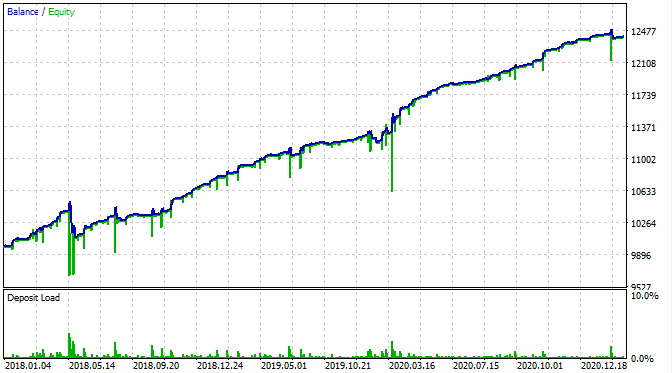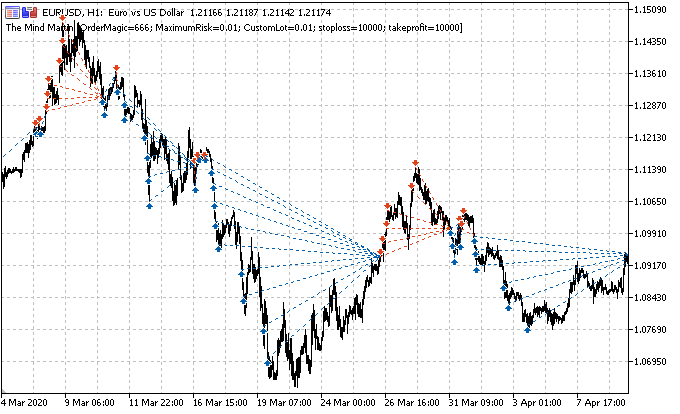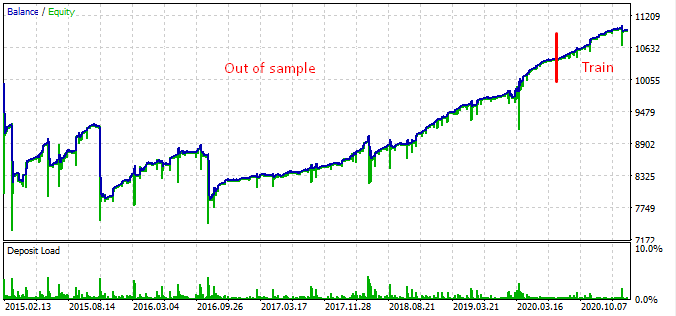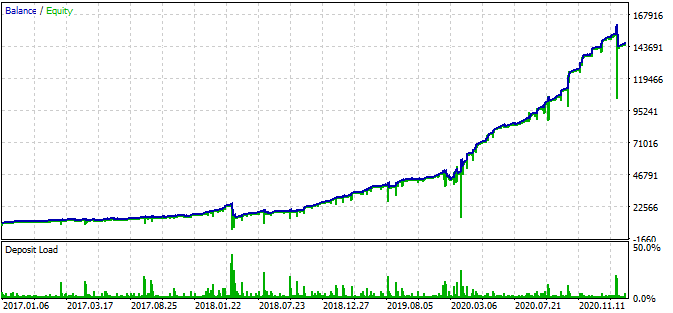`GRID_COEFFICIENTS = np.linspace(1, 5, num= GRID_SIZE)`
```array([1.        , 1.44444444, 1.88888889, 2.33333333, 2.77777778,
3.22222222, 3.66666667, 4.11111111, 4.55555556, 5.        ])
```

## 量化课程

移动端课程